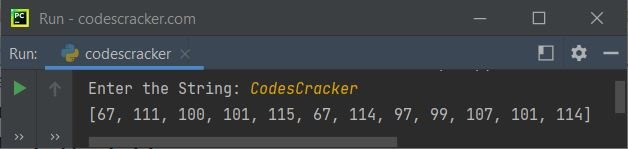# bytes in Python

bytes in Python are of the binary sequence type. Here is the list of three binary sequence types available in Python:

1. bytes
2. bytearray
3. memoryview

bytes are the fundamental inbuilt types used in binary data manipulation, such as bytearray. Unlike bytearray, bytes objects are immutable sequences of single bytes, which are basically arrays of octets, used for storing the data but not the text.

Note: bytes objects are similar to string objects. The difference is: values of bytes literals come with a "b" prefix. For example: b'codes', b'codescracker dot com', and so on.

Important: Only ASCII characters are allowed in bytes literals.

## Creating bytes literals in Python

A "bytes" literal can be created in one of the following ways:

1. using single quotes
2. using double quotes
3. using three single quotes
4. using three double quotes

The b prefix is required in all the cases.

## Python bytes example

Here is an example of bytes in Python. This program generates bytes objects in all of the ways described above. This program creates a bytes object and prints the value along with the type using the type() method.

```x = b'allows embedded "double" quotes'
print(x)
print(type(x))

x = b"allows embedded 'single' quotes"
print(x)
print(type(x))

x = b'''three single quotes'''
print(x)
print(type(x))

x = b"""three double quotes"""
print(x)
print(type(x))```

The snapshot given below shows the sample run of the above Python program, demonstrating the creation of bytes in Python:### ASCII Code Representing the Character of a bytes Object in Python

This program is created to receive a string input from the user at run-time and print the ASCII code corresponding to all the characters available in the given string:

```print("Enter the String: ", end="")
s = input()

s = bytes(s, "utf-8")
print(list(s))```

The snapshot given below shows the sample run with user input, say "CodesCracker" as a string to list and print the ASCII values of all characters:## Python bytes Object Slicing

Just like a list, a bytes object can also be sliced to get some part of the bytes object value. Here is an example of bytes slicing.

```x = b"python programming"
print(x)
print(x[0:])
print(x[-18:])
print(x[0:6])
print(x[7:14])```

The 0th index refers to the index of the very first element. Adding the minus (-) sign before the index refers to the native or backward indexing. That is, -1 refers to the index of the very first element from last, whereas -18 refers to the 18th element's index from last. The output produced by the above program should be:

```b'python programming'
b'python programming'
b'python programming'
b'python'
b'program'```

Note: For more information on slicing, see the separate tutorial on list .You'll find all the information there.

To convert a bytes object to a string object, refer to the Python bytes to string program.

Python Online Test

« Previous Tutorial Next Tutorial »